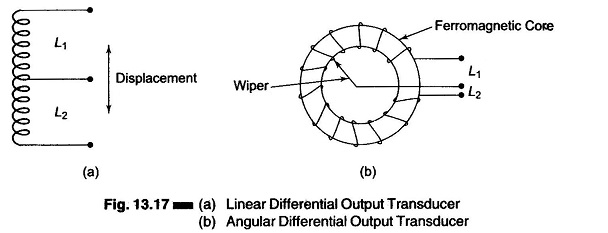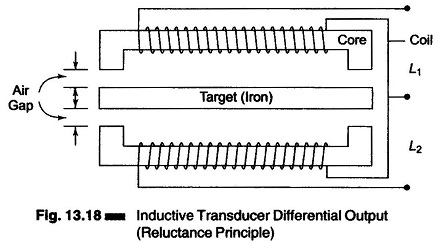## Differential Output Transducer:

The Differential Output Transducer consists of a coil which is divided into two parts, as shown in Figs. 13.17(a) and (b).(Inductive transducers using self inductance as a variable use one coil, while those using mutual inductance as a variable use multiple coils.)

Normally the change in self inductance, ΔL, for inductive transducers, (working on the principle of change of self inductance) is not sufficient for detection of subsequent stages of the instrumentation system.

However, if successive stages of the instrument respond to ΔL or ΔM, rather than L + ΔL, or M + ΔM, the sensitivity and accuracy will be much higher.

The transducers can be designed to provide two outputs, one of which represents inductance (self or mutual) and the other the decrease in inductance (self or mutual). The succeeding stages of the instrumentation system measure the difference between these outputs. This is known as differential output.

### Advantages of Differential Output Transducer:

1. Sensitivity and accuracy are increased.
2. Output is less affected by external magnetic fields.
3. Effective variations due to temperature changes are reduced.
4. Effects of change in supply voltages and frequency are reduced.

In response to a physical signal (which is normally displacement), the inductance of one part increases from L to L + ΔL, while that of the other part decreases from L to L – ΔL. The change is measured as the difference of the two, resulting in an output of 2 ΔL instead of ΔL, when one winding is used. This increases the sensitivity and also eliminates error.Inductive transducers using the change in the number of turns to cause a change in the self inductance are shown in Fig. 13.17.

Figure 13.17(a) is used for measurement of linear displacement using an air cored coil.

Figure 13.17(b) is used for the measurement of angular displacement using an iron cored coil.

Figure 13.18 shows an inductive transducer giving a differential output. The output represents a change of self inductance due to change of reluctance. (This inductive transducer also works on the principle of change of self inductance of the two coils with change in reluctance of the path of the magnetic circuit. The target as well as cores on which the coil is wound are made up of iron.)

Scroll to Top